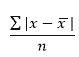## Statistical functions: avedev, average, averagea, countblank, countif

#### Excel Function AVEDEV

The AVEDEV() function calculates the average deviation of a given set of values.

The average deviation is the deviation of set of values from their average value.

The formula for calculating the AVEDEV for n number of values represented by x and average value of xA maximum of 255 arguments can be supplied in this function.

 Values AVEDEV() Formula 1,2,3,4 1 =AVEDEV(1,2,3,4) 5,56,6,90 33.75 =AVEDEV(5,56,6,90) 34 0 =AVEDEV(L7) 21 6.5 =AVEDEV(L7:L8) 45 14.16 =AVEDEV(L7:L9,4,5)

#### Excel Function AVERAGE

The AVERAGE() function is used to calculate the average value of a set of numbers.

This function can take a maximum of 255 values.

The syntax is :

AVERAGE(num1,num2,�num255)

 Values AVERAGE() Formula 1,2,3,4 2.5 =AVERAGE(1,2,3,4) 5,56,6,90 39.25 =AVERAGE(5,56,6,90) 34 34 =AVERAGE(L22) 21 27.5 =AVERAGE(L22:L23) 45 30 =AVERAGE(L22:L24,20)

#### Excel Function AVERAGEA

The AVERAGEA() function is also used to calculate the average of a set of values.

The difference from AVERAGE() function is that, AVERAGEA() can also include Boolean and number values

represented as text.

 Values AVERAGEA() Formula True 0.5 =AVERAGEA(L33:L34) False 0 =AVERAGEA(L34) 34 17 =AVERAGEA(L34:L35) 21 33.3333333333333 =AVERAGEA(L35:L36,"45") 45 33.3333333333333 =AVERAGEA(L35:L37)

#### Excel Function COUNTBLANK

The COUNTBLANK() function is used to calculate the number of blank cells within a range.

The cells containing text,numbers or any other symbols are not counted.

Even the white space is also not considered as blanks.

 Values COUNTBLANK() Formula Remarks Eating 0 =COUNTBLANK(L45) Study 0 =COUNTBLANK(L45:L47) 1 =COUNTBLANK(L47:L48) White space 1 =COUNTBLANK(L47:L49) Blank cell 45 1 =COUNTBLANK(L45:L49)

#### Excel Function COUNTIF

The COUNTIF() function is used to count the number of cells within a range that satisfies a certain criteria.

This function returns a number that represents cell count matching the supplied criteria.

The syntax is as follows:

COUNTIF(range,criteria)

 Values COUNTIF() Formula Remarks Eating 0 =COUNTIF(L57:L58,"Vaishu") Study 0 =COUNTIF(L57:L58,"nag") Not case sensitive 0 =COUNTIF(L57:L59,"") White space 0 =COUNTIF(L57:L61,1) Blank cell 45 1 =COUNTIF(L57:L61," ")